### Registrar: memory operator for Evolutionary Algorithms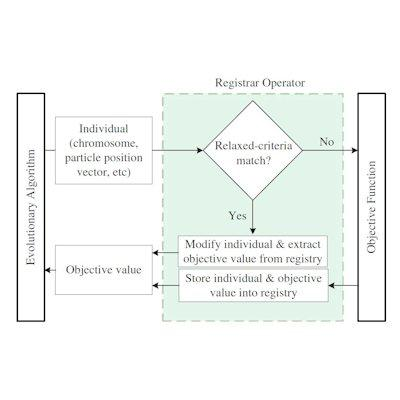## Introduction

xlOptimizer fully implements the Registrar, a memory operator which increases the performance of Evolutionary Algorithms (EA) in case of expensive function evaluations . The Registrar does not approximate the objective function. Thus, it does not require validation, error compensation or reliability indices. Instead, it records all accurately evaluated solutions, hence the name, and selectively replaces the solutions requested by the EA with similar ones taken from the registry, bypassing the function evaluation. Unlike other methods that use external memory to increase genetic diversity, this simple implementation encourages revisits in order to save evaluations in an aggressive manner. It also features complete memory and is indifferent to the quality of solutions that are reused. Both genotypic and phenotypic similarity criteria can be used. Significant increase in performance is observed that can reach a factor of twenty in 10-dimensional problems and a factor of ten in 30-dimensional problems. This increase is present even at the early stages of evolution, in accordance with the Birthday Problem of probability.

## How does it work?

The Registrar operator is a fully-encapsulated simple piece of code that is inserted in between the EA and the Objective Function, as shown schematically in the figure. When a function evaluation is requested, the operator scans the registry for possible matches based on current similarity criteria. If one or more matches are found, the Registrar replaces the individual by its best match. This replacement is reflected to the actual population of the EA. The Registrar also extracts the corresponding objective value and returns it to the EA, bypassing the subroutine. Thus, a function evaluation is saved. If no satisfactory matches are found, the call is forwarded to the subroutine for evaluation. Then, the result is intercepted before it is returned to the EA and stored into the registry, together with the associated individual. The memory requirements for storing the individuals and the corresponding objective values are easily accommodated by modern personal computers. Note that the Registrar is fully deterministic.

## Mathematical formulation

The Registrar can be implemented with match criteria that are formed in genotypic terms. If two chromosomes cA  and cB differ in (at most) a certain number of corresponding bits, then there is a “match”. The number of different corresponding bits D is evaluated as: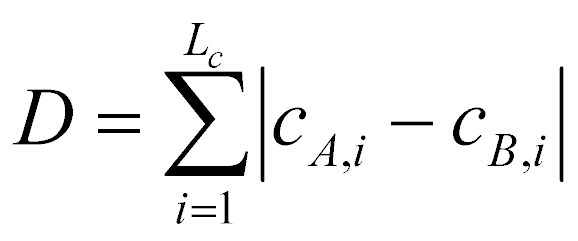where, L= chromosome length, cA,i  and cB,i  = ith bit of chromosome cA  and cB , respectively. Thus, the normalized relaxed-match criterion can be expressed as: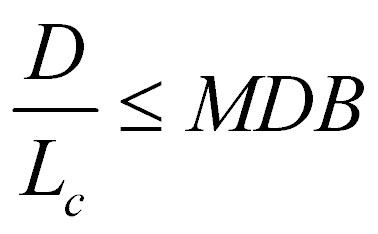where, MDB = maximum different bits fraction. The Registrar can also be implemented with match criteria that are formed in phenotypic terms (although this is not recommended for Genetic Algorithms). Herein, the “distance”   between two chromosomes  cA  and cB is defined as the sum of the normalized “city block distances” of their corresponding phenotypic values: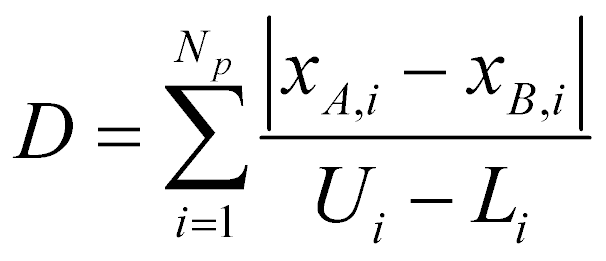where, Np = number of variables, xA,i  and xB,i  = ith phenotypic value of individual cA and cB , respectively, and Ui and Li = upper and lower bound of variable i, respectively. When D is smaller than a certain threshold, then there is a match. Thus, the criterion can be written as: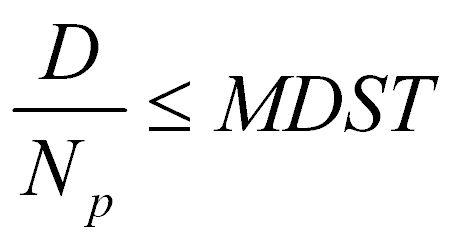where, MDST = mean distance. An adaptive reduction method of MDB and MDST should be used. Evolution begins with initial MDB and MDST values; whenever the rate of virtual over total evaluations exceeds a maximum rate MR, we multiply the current MDB and MDST values with MLT<1 to reduce them, and thus make the match criteria more strict.

## Options in xlOptimizer

The Registrar is available in most Evolutionary Algorithms as an option. For example, in Genetic Algorithms the following form is available: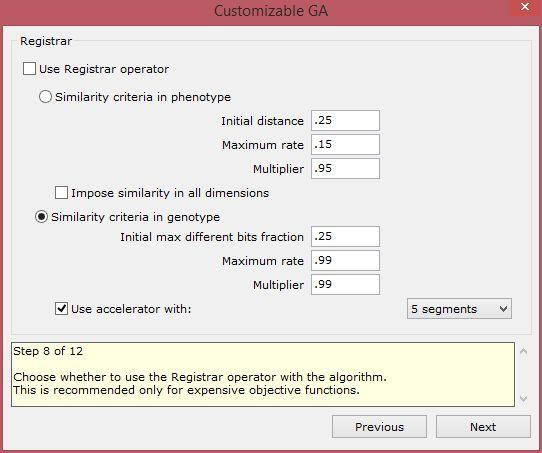Check 'Use Registrar operator' in order to activate the operator. You can choose whether to define the match criteria in the phenotype (this is the only option for EAs that use real numbers) or in the genotype (this is recommended for Genetic Algorithms). The other options are described in the mathematical formulation section. The 'accelerator' is an operator which accelerates the comparison of the bits between chromosomes. This is always recommended.